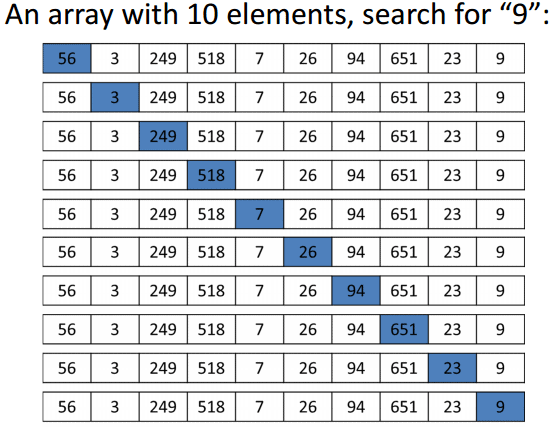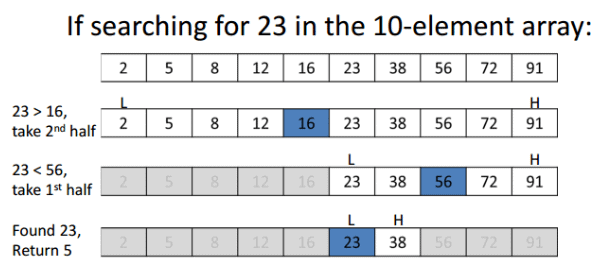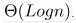Courses

# Searching Computer Science Engineering (CSE) Notes | EduRev

## Computer Science Engineering (CSE) : Searching Computer Science Engineering (CSE) Notes | EduRev

The document Searching Computer Science Engineering (CSE) Notes | EduRev is a part of the Computer Science Engineering (CSE) Course GATE Computer Science Engineering(CSE) 2022 Mock Test Series.
All you need of Computer Science Engineering (CSE) at this link: Computer Science Engineering (CSE)

## Linear Search

Problem: Given an array arr[] of n elements, write a function to search a given element x in arr[].

A simple approach is to do linear search, i.e

• Start from the leftmost element of arr[] and one by one compare x with each element of arr[]
• If x matches with an element, return the index.
• If x doesn’t match with any of elements, return -1.

Example:// Linearly search x in arr[].  If x is present then return its
// location,  otherwise return -1
int search(int arr[], int n, int x)
{
int i;
for (i=0; i<n; i++)
if (arr[i] == x)
return i;
return -1;
}

The time complexity of above algorithm is O(n).

Linear search is rarely used practically because other search algorithms such as the binary search algorithm and hash tables allow significantly faster searching comparison to Linear search.

## Binary Search

Given a sorted array arr[] of n elements, write a function to search a given element x in arr[].

A simple approach is to do linear search. The time complexity of above algorithm is O(n). Another approach to perform the same task is using Binary Search.

Binary Search: Search a sorted array by repeatedly dividing the search interval in half. Begin with an interval covering the whole array. If the value of the search key is less than the item in the middle of the interval, narrow the interval to the lower half. Otherwise narrow it to the upper half. Repeatedly check until the value is found or the interval is empty.

Example:The idea of binary search is to use the information that the array is sorted and reduce the time complexity to O(Logn).

We basically ignore half of the elements just after one comparison.

1. Compare x with the middle element.
2. If x matches with middle element, we return the mid index.
3. Else If x is greater than the mid element, then x can only lie in right half subarray after the mid element. So we recur for right half.
4. Else (x is smaller) recur for the left half.

Recursive implementation of Binary Search

#include <stdio.h>

// A recursive binary search function. It returns location of x in
// given array arr[l..r] is present, otherwise -1
int binarySearch(int arr[], int l, int r, int x)
{
if (r >= l)
{
int mid = l + (r - l)/2;

// If the element is present at the middle itself
if (arr[mid] == x)  return mid;

// If element is smaller than mid, then it can only be present
// in left subarray
if (arr[mid] > x) return binarySearch(arr, l, mid-1, x);

// Else the element can only be present in right subarray
return binarySearch(arr, mid+1, r, x);
}

// We reach here when element is not present in array
return -1;
}

int main(void)
{
int arr[] = {2, 3, 4, 10, 40};
int n = sizeof(arr)/ sizeof(arr);
int x = 10;
int result = binarySearch(arr, 0, n-1, x);
(result == -1)? printf("Element is not present in array")
: printf("Element is present at index %d", result);
return 0;
}

Output:
Element is present at index 3

Iterative implementation of Binary Search

#include <stdio.h>

// A iterative binary search function. It returns location of x in
// given array arr[l..r] if present, otherwise -1
int binarySearch(int arr[], int l, int r, int x)
{
while (l <= r)
{
int m = l + (r-l)/2;

// Check if x is present at mid
if (arr[m] == x)
return m;

// If x greater, ignore left half
if (arr[m] < x)
l = m + 1;

// If x is smaller, ignore right half
else
r = m - 1;
}

// if we reach here, then element was not present
return -1;
}

int main(void)
{
int arr[] = {2, 3, 4, 10, 40};
int n = sizeof(arr)/ sizeof(arr);
int x = 10;
int result = binarySearch(arr, 0, n-1, x);
(result == -1)? printf("Element is not present in array")
: printf("Element is present at index %d", result);
return 0;
}

Output:
Element is present at index 3

Time Complexity:
The time complexity of Binary Search can be written as

T(n) = T(n/2) + c

The above recurrence can be solved either using Recurrence T ree method or Master method. It falls in case II of Master Method and solution of the recurrence isAuxiliary Space: O(1) in case of iterative implementation. In case of recursive implementation, O(Logn) recursion call stack space.

Offer running on EduRev: Apply code STAYHOME200 to get INR 200 off on our premium plan EduRev Infinity!

## GATE Computer Science Engineering(CSE) 2022 Mock Test Series

131 docs|167 tests

,

,

,

,

,

,

,

,

,

,

,

,

,

,

,

,

,

,

,

,

,

;